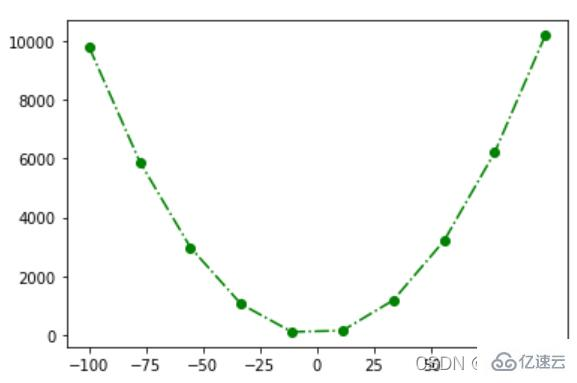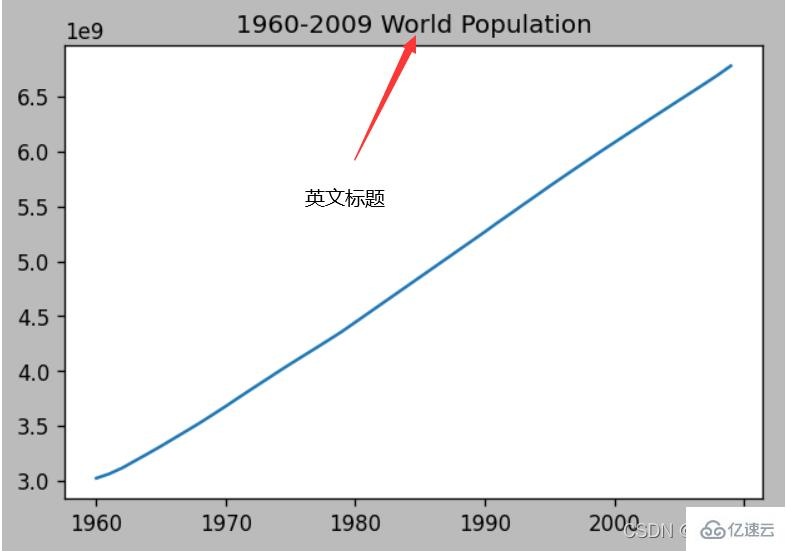# Python中如何使用plot()函数画图### 一、plot()函数的认识

matplotlib图的组成：

• Figure （画布）

• Axes (坐标系)

• Axis (坐标轴)

• 图形（plot(),scatter(),bar(),...)

• Title, Labels, ......

`plt.plot(x, y, fmt='xxx', linestyle=, marker=, color=, linewidth=, markersize=, label=, )`

（1）linestyle：此字段是线的样式，参数形式：字符串

linestyle(线的样式)
 linestyle参数 线形 '-' 实线 '--' 虚线 '-.' 点划线 ':' 点虚线 ' ' 无线

（2）linewidth：此参数是线的粗细，粗细程度和所定数值大小有关，参数形式：数值

（3）marker：点的样式，字符串

marker（点的样式）
 marker 标记点 '.' 店 ',' 像素 '^' 'v' '>' '<' 上下左右三角形 '1' '2' '3' '4' 上下左右三叉线 'o' 圆形 's' 'D' 方形 'p' 五边形 'h' 'H' 六边形 '*' 五角星 '+' 'x' 十字交叉 '_' 横线 ' '

（4）markersize：点的大小，参数形式：数值

（5）color：调节线条还有点的颜色 ，字符串，参数形式字符串

color（点、线颜色）
 字符串 color 'r' 红 'g' 绿 'b' 蓝 'y' 黄 'c' 青 'm' 品 'k' 黑 'w' 白

（6）label：图例，legend文字

### 二、plot()函数基本运用

```import matplotlib.pyplot as plt
plt.plot()```

```# 导入包
import matplotlib.pyplot as plt
import numpy as np
# 构造数据
# 位置 （2维：x,y一一对应）
x = np.linspace(0, 2 * np.pi, 200)  # 从0到2pi的200个值
y = np.sin(x)                       # 从sin(0)到sin(2pi)的200个值
# 颜色（0维）
c = 'red'
c = 'r'
c = '#FF0000'
# 大小（0维）: 线宽
lw = 1```

```# 生成一个Figure画布和一个Axes坐标系
fig, ax = plt.subplots()
# 在生成的坐标系下画折线图
ax.plot(x, y, c, linewidth=lw)
# 显示图形
plt.show()``````import matplotlib.pyplot as plt
x = [1,2,3]
y = [1,2,3]
y = x
plt.plot(x,y,linestyle=':', linewidth=1, marker='d', markersize=10, label='1234')
plt.legend()``````import matplotlib.pyplot as plt
import numpy as np
x = np.linspace(-100,100,10)
y = x**2 + 2*x +1
plt.plot(x,y,'g-.o')``````#导入包
import matplotlib.pyplot as plt
import numpy as np
import pandas as pd

#使用linspace（）方法构成数据
x = np.linspace(0, 2 * np.pi, 50)  #
y1 = np.sin(x)
y2 = np.cos(x)
#转化数据形式
df = pd.DataFrame([x,y1,y2]).T
#对列重新命名
df.columns = ['x','sin(x)','cos(x)']
#数据写入图像，命名图例
plt.plot(df['x'],df['sin(x)'],label='sin(x)')
plt.plot(df['x'],df['cos(x)'],label='cos(x)')
plt.legend()```### 三、plot()函数数据可视化画图以及图元基本参数设置

```import pandas as pd
datafile = r'world_population.txt'  # 打开文件```import matplotlib.pyplot as plt
# 画布
fig = plt.figure(figsize=(6,4),  # inches
dpi=120, # dot-per-inch
facecolor='#BBBBBB',
frameon=True, # 画布边框
)
plt.plot(df['year'],df['population'])

# 标题
plt.title("1960-2009 World Population")```

```# 设置中文字体
plt.rcParams['font.sans-serif'] = 'SimHei'  # 设置字体为简黑（SimHei）
plt.rcParams['font.sans-serif'] = 'FangSong'  # 设置字体为仿宋（FangSong）``````import matplotlib.pyplot as plt

# 设置中文字体
plt.rcParams['axes.unicode_minus'] = False    # 不使用中文减号
plt.rcParams['font.sans-serif'] = 'FangSong'  # 设置字体为仿宋（FangSong）

# 画布
fig = plt.figure(figsize=(6,4),  # inches
dpi=120, # dot-per-inch
facecolor='#BBBBBB',
frameon=True, # 画布边框
)
plt.plot(df['year'],df['population'],'b:o',label='人口数')

# 中文标题
plt.title("1960-2009 世界人口")

# 字体字典
font_dict=dict(fontsize=8,
color='k',
family='SimHei',
weight='light',
style='italic',
)

# X轴标签
plt.xlabel("年份", loc='center', fontdict=font_dict)   # loc: 左中右 left center right

# Y轴标签
plt.ylabel("人口数",loc='top', fontdict=font_dict)  # loc: 上中下 top center bottom

# X轴范围
plt.xlim((2000,2010))  # X轴的起点和终点

# Y轴范围
plt.ylim(6e9,7e9) # Y轴的起点和终点

# X轴刻度
plt.xticks(np.arange(2000,2011))

# X轴刻度
plt.yticks(np.arange(6e9,7e9+1e8,1e8))

# 图例
plt.legend()
# plt.legend(labels=['人口'])

# 网格线
plt.grid(axis='y')  # axis: 'both','x','y'```